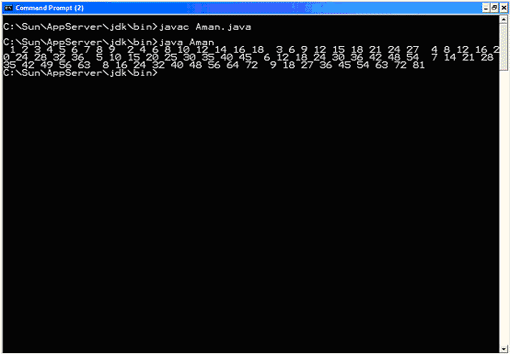## Core Java

Java Evolution
Overview of Java Language
Constant, Variable and Datatype
Operator and Expression
Decision making, Branching and Looping
Class Object and Method
Array and String
Inheritance
Package
 1) It defines a block of code to be executed as long as a particular condition is met. The     condition is tested at the end of each iteration. It always guaranteed at least one pass     through a do-while loop. syntax do { statements; } while (condition); where condition represents a binary expression that, if true, permits another iteration of the loop to be performed. If no longer true, processing continues at the next statement. The braces may be omitted if the loop consists of a single statement. This would constitute a "single statement do-while loop". It can be used in place of nearly any while loop as shown by these examples: Example 1: Counting to 10 without a local variable int i = 1; do { System.out.println(i); i++; } while (i <= 10); Loop control variable i is initialized outside the loop. Within the loop, the current value of i is displayed and i is incremented. At the end of each iteration, the value of i is tested to determine if it is still less than or equal to 10. If so, the body of the loop is repeated. Otherwise processing will jump to the next statement. Example 2: An endless loop do { System.out.println("I won't end"); } while(true); Example 3: A small program using nested do-while loops to generate a 9 x 9 multiplication table import java.io.*; public class Aman { public static void main(String[] args) { // Initialize the row number. int row = 1; // This outer loop generates one row of the multiplication // table during each iteration. do { // Initialize the column number. int column = 1; // This inner loop generates one column of the current row // of the multiplication table during each iteration. do { // If a one digit number is about to be displayed, preceed it // with four spaces. Otherwise, preceed it with three spaces. if ((row * column) < 10) { System.out.print(" "); } else { System.out.print(" "); } // Display the number. System.out.print((row * column)); // Increment the column number. column++; } while (column <= 9); // End the current line. System.out.print(" "); // Increment the row number. row++; } while (row <= 9); } } Output: